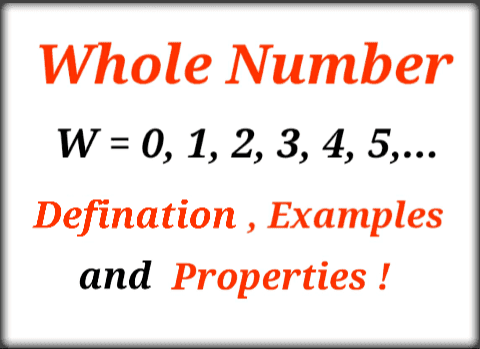###### Latest Topics## Whole Number Properties

On the number line, the set of natural numbers and the set of whole numbers may be represented as illustrated below. The natural numbers are represented by all positive integers or integers on the right-hand side of 0, while the whole numbers are represented by all positive integers plus zero. On the number line, both sets of numbers may be represented as follows:

# Number Line with Whole and Natural Numbers

Whole Number Properties:-

Addition, subtraction, multiplication, and division are the four fundamental operations on whole numbers. This lead to the four major characteristics of whole numbers mentioned below:

Closure Property

Associative Property

Commutative Property

Distributive PropertyA whole number is always the sum and product of two whole numbers. For instance, 7 + 3 Equals 10 (whole number), and 7x 2 = 14 (whole number).

Property of Association:-

Even if the order of the numbers is altered, the sum or product of any three whole numbers stays the same. When we put the following integers together, we obtain the same result:

10 + (10 + 12) = (10 + 10) + 12 = (10 + 12) + 10 = 32.

Similarly, no matter how the numbers are arranged, we obtain the same result when we multiply them:

3 × (2 × 2) = (3 × 2) × 2 = 12.

Property of Commutation (Applying commutative law on whole numbers):-

Even if the order of the numbers is reversed, the sum and product of two whole numbers remain the same. This characteristic says that changing the order of addition has no effect on the sum's value. According to the commutative property a + b = b + a, let 'a' and 'b' be two whole integers.

Example:

If a = 10 and b = 20, for example, 10 + 20 = 30 = 20 + 10. It implies that when you put two whole numbers together, you get a closed number. This feature also applies to multiplication, but not to division or subtraction.

For instance:

6 × 9 = 54 and 9 × 6 = 54.

Associative identity: (Applying associative law on whole numbers)

Adding a whole number to 0 does not change its value,

If x is a whole number, a + 0 = 0 + a = a. For instance, 5 + 0 Equals 5.

Multiplicative identity:

When a whole number is multiplied by one, the value stays the same, thus if a is a whole number, a.1 = a = 1.a. As an example. 5x1=5

Property of Distribution (Applying distributive law on whole numbers):-

The multiplication of a whole number is spread across the total of the whole numbers. According to this property, if two numbers, say a and b, are multiplied by the same number c and then added, the sum of the two numbers may be multiplied by c to obtain the same result. This scenario is represented by the equation

a × (b + c) = (a × b) + (a × c).

Example:

Let a = 10, b = 10 and c = 2 ⇒ 10 × (10 + 2) = 120 and (10 × 20) + (10 × 7) = 200 + 70 = 270. The same property applies for subtraction too.

An online platform that provides comprehensive explanations of whole numbers:

By using our e-learning platform, students may get a thorough understanding of whole numbers and other math topics. Learners are exposed to a wide range of video lectures, as well as 2-D and 3-D animations and simulations. It also contains extensive textual content, PowerPoint presentations, assignments, and supporting illustrations related to a subject. Choose any subject from us or one of our learning partners that fit your budget.